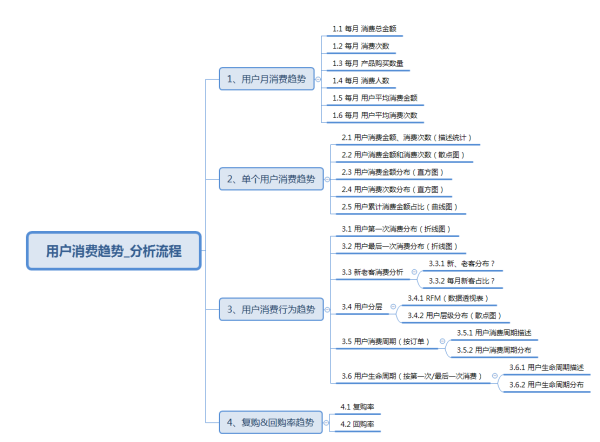• 运筹帷幄，增长黑客，行业洞悉，问题精准定位使营业额翻他个n倍；
• 工具必须得用高端的啊，只靠sql和excel就太low了，必须用python + R各种分析；
• 绘图只用黑白曲线不行吧？必须得逼格高点，搞个BI报表美化下吧

• 工作内容：大多数情况下是解决实际问题的

1）具体业务需求，比如运营活动效果分析，产品新功能上线前用ABtest进行对比分析

2）专题分析，比如留存分析，用户转化，新老用户差异等等

• 工具使用：偏向业务的数据分析师主要工具还是excel和sql ，如果会用python有可能会更加便捷
• 分析方法：简单来讲就是细分、对比、找特征+相关性，具体怎么细分，用哪些模型找相关性，要根据具体业务场景确定。不论是数据分析、数据挖掘还是机器学习，大方向都是在寻找数据间的特征和相关性，最后让数据真正服务于业务

• 分析目的：

• 分析流程：

• 数据来源：一份国外网站的产品销售记录
• 分析工具：Python

python库：pandas、numpy、matplotlib

• 理解字段：
user_id 用户ID
order_dt: 消费日期
order_products: 消费产品数量
order_amount: 消费金额
• 导入python库：
import pandas as pd
import numpy as np
#加载数据可视化包
import matplotlib.pyplot as plt

#可视化显示在页面 jupyter专属 %内置
%matplotlib inline
#更改设计风格
plt.style.use('ggplot')
• 导入数据
# txt格式用read_table 如果有多个字符串 sep ='\s+' 正常csv用的是 ','
# parse_dates='order_dt' 将购买日期转换为时间格式，date_parser='%Y%m%d'具体时间格式
df = pd.read_table('CDNOW_master.txt',names = columns,sep= '\s+')

parse_dates='order_dt', date_parser='%Y%m%d'

• 查看前五行数据
df.head()
• 查看数据结构类型：共69659行、4列，前3列为整数类型，第4列为浮点型，各列行数与总行数69659一致，说明数据无空值
df.info()
• 描述性统计
df.describe()

1）大部分订单只消费了少量商品（平均2.4件），中位数为2件，说明有一定极值干扰拉高了平均值

2）用户的消费金额比较稳定，平均消费35元，中位数在25元，同样说明有一定极值干扰

• 3.1 选择子集（本数据集不需要选择子集）
• 3.2 列名重命名
#命名列名
columns = ['user_id','order_dt','order_products','order_amount']
• 3.3 缺失数据处理（已知无空值）
• 3.4 数据类型转换
# to_datetime  日期转换函数
# format 日期格式：Y代表四位年份 y两位年份 M分钟 m月份 d天
df['order_dt']= pd.to_datetime(df.order_dt,format="%Y%m%d")
• 3.5 数据排序（排序意义不大，暂不排序）
• 3.6 异常值处理（由描述性统计部分可知，暂无异常值）

#新增一列月份month   [M]代表月份  values将其转换成数组 否则会报错
df['month'] = df.order_dt.values.astype('datetime64[M]')

### 1. 用户月消费趋势分析

• 1.1 每月 消费总金额
#按月分组
grouped_month = df.groupby('month')

#月消费总金额 = 按月分组.消费金额.sum()
order_month_amount = grouped_month.order_amount.sum()
#查看前五行
order_month_amount.head()
# 可视化
order_month_amount.plot()

• 1.2 每月 消费次数
#月订单数
grouped_month.user_id.count().plot()

• 1.3 每月 产品购买数量
#月产品购买数量
grouped_month.order_products.sum().plot()

• 1.4 每月 消费人数

"""

duplicates去重；

"""
grouped_month.user_id.apply(lambda x:len(x.drop_duplicates())).plot()
#其他方法
#df.groupby(['month','user_id']).count().reset_index()

• 1.5 每月 用户平均消费金额
#每月用户平均消费金额 = 月分组.总金额/用户数
((grouped_month.order_amount.sum())/(grouped_month.user_id.apply(lambda x:len(x.drop_duplicates())))).plot()

• 1.6 每月 用户平均消费次数
#每月用户平均消费次数的趋势  消费总次数/消费用户数
((grouped_month.user_id.count())/(grouped_month.user_id.apply(lambda x:len(x.drop_duplicates())))).plot()

### 2.单个用户消费趋势分析

• 2.1 用户消费金额、消费次数的描述统计
grouped_user = df.groupby('user_id')
grouped_user.sum().describe()

• 2.2 用户消费金额和消费次数的散点图
grouped_user.sum().plot.scatter(x = 'order_amount',y = 'order_products')

grouped_user.sum().query('order_amount < 4000').plot.scatter(x = 'order_amount',y = 'order_products')

• 2.3 用户消费金额的分布图
# 用户消费金额的分布图 （直方图） bins = 20 将x值平均分成20份
grouped_user.sum().order_amount.hist(bins = 20)

• 2.4 用户消费次数的分布图
#调整图表尺寸
plt.figure(figsize=(8,5))
#为避免极值干扰结果，中间使用query函数过滤掉极值
grouped_user.count().query('order_products < 100').order_products.hist(bins = 40)

（由2.1的描述统计表 消费产品数平均值为7，标准差为17 所以上限=7+17*5 约为100）

• 2.5 用户累计消费金额占比（百分之多少的用户占了百分之多少的消费额）
# 按用户分组并求和,再对订单金额进行排序(默认从小到大),最后通过匿名函数对每一行进行累计求和占比
user_cumsum = grouped_user.sum().sort_values('order_amount').apply(lambda x:x.cumsum()/ x.sum())
user_cumsum.order_amount.cumsum()/user_cumsum.order_amount.sum()
#对计算后的用户消费金额重新排序后绘图
user_cumsum.reset_index().order_amount.plot()

3.用户消费行为

• 3.1 用户第一次消费分布（首购）
plt.figure(figsize=(8,5))
grouped_user.min().order_dt.value_counts().plot()

• 3.2 用户最后一次消费
grouped_user.max().order_dt.value_counts().plot()

• 3.3 新老客消费分析

3.3.1多少用户仅消费了一次？

user_life = grouped_user.order_dt.agg(['min','max'])
user_life.head()
# 最早消费时间与最后消费时间一致表示只消费了一次，显示为True
(user_life['min'] == user_life['max']).value_counts()

True 12054
False 11516
dtype: int64

3.3.2 每月新客占比？

#按月份和用户ID分组
grouped_month_user=df.groupby(['month','user_id'])

#将 当月用户订单日期最小值 与 用户订单日期最小值 联结
tmp=grouped_month_user.order_dt.agg(['min']).join(grouped_user.order_dt.min())

# 判断用户当月订单日期最小值是否与用户订单日期最小值相等，新建字段old,old代表老客户
tmp['old']=(tmp['min']==tmp.order_dt)

# 重置索引列,并按月分组,作新客占比折线图
tmp.reset_index().groupby('month').old.apply(lambda x: x.sum()/x.count()).plot()

• 3.4 用户分层

3.4.1 RFM，

#新建数据透视表
RFM = df.pivot_table(index = 'user_id',
values= ['order_products','order_amount','order_dt'],
aggfunc = {'order_dt':'max',
'order_amount':'sum',
'order_products':'sum'
})
RFM.head()
#定义参数R
RFM['R']=-(RFM.order_dt - RFM.order_dt.max()) / np.timedelta64(1,'D')
RFM.rename(columns = {'order_products':'F','order_amount':'M'},inplace = True)
R:最后一次消费距离统计日期之间的天数，天数越小代表用户价值越高
F:消费总商品数，越大用户价值越高
M:消费总金额，越大用户价值越高
#匿名函数，分别计算R F M 与平均值之间的差
RFM[['R','F','M']].apply(lambda x:x-x.mean())
# 定义用户八个层级，根据业务定义打分
def rfm_func(x):
level = x.apply(lambda x:'1' if x>=0 else '0')
#label标签,  字符串拼接 '+'
label = level.R + level.F + level.M
d = {
'111':'重要价值客户',
'011':'重要保持客户',
'101':'重要挽留客户',
'001':'重要发展客户',
'110':'一般价值客户',
'010':'一般保持客户',
'100':'一般挽留客户',
'000':'一般发展客户'
}
result = d[label]
return result
RFM['label'] = RFM[['R','F','M']].apply(lambda x:x-x.mean()).apply(rfm_func,axis =1)


#计算八个层级客户的R、F、M和
RFM.groupby('label').sum()

#计算各层级客户的数量分布
RFM.groupby('label').count()

#各类客户占比
user_c = RFM.groupby('label').count()
#避免压缩为椭圆
plt.axis('equal')
#定义标签列表
labels = ['一般价值客户','一般保持客户','一般发展客户','一般挽留客户','重要价值客户','重要保持客户','重要发展客户','重要挽留客户']
#绘制饼图  按月对客户数计数，
plt.pie(user_c['M'],
autopct='%3.1f%%',
labels = labels,
pctdistance=0.9,
labeldistance = 1.2,
startangle = 15)
# 正常显示中文标签plt.rcParams   思黑字体['SimHei']
plt.rcParams['font.sans-serif']=['SimHei'] 

• 3.4.2 用户状态分层（新、老、活跃、流失）
# 数据透视表，空值填充为0
pivoted_counts = df.pivot_table(index = 'user_id',
columns = 'month',
values = 'order_dt',
aggfunc = 'count').fillna(0)
pivoted_counts.head()
#消费过的为1 ，没消费过的为0
data_purchase = pivoted_counts.applymap(lambda x: 1 if x > 0 else 0)

-若之前是未注册，则依旧为未注册
-若之前有消费，则为流失/不活跃
-其他情况，为未注册

-若是第一次消费，则为新用户
-若之前有过消费，则上个月为不活跃，则为回流
-若上个月为未注册，则为新用户
-除此之外，为活跃
#用户状态
def active_status(data):
status = []
for i in range(18):
#若本月没有消费
if data[i] == 0:
if len(status) > 0:
if status[i-1] == 'unreg':
status.append('unreg')
else:
status.append('unactive')
else:
status.append('unreg')
#若本月消费
else:
if len(status) == 0:
status.append('new')
else:
if status[i-1] == 'unactive':
status.append('return')
elif status[i-1] == 'unreg':
status.append('new')
else:
status.append('active')
# 这里需要对返回的值进行转换，将列表转为Series
return pd.Series(status, index = pivoted_counts.columns)

purchase_stats = data_purchase.apply(active_status,axis=1)
# df.rename(columns={ df.columns: "new name" }, inplace=True)
# purchase_stats.rename(columns={purchase_stats.columns:data_purchase.columns})
purchase_stats.head()
#每个月活跃用户的计数
purchase_status_ct = purchase_stats.replace('unreg',np.NaN).apply(lambda x : pd.value_counts(x))
purchase_status_ct
#将 NaN 填充为0 ，转置
purchase_status_ct.fillna(0).T
#面积图 plot.area()
purchase_status_ct.fillna(0).T.plot.area()
#计算各消费状态层级用户占比
purchase_status_ct.fillna(0).T.apply(lambda x:x/x.sum(),axis = 1)

-活跃用户，即持续消费的用户，对应的是消费运营的质量

-回流用户，之前不消费本月才消费，对应的是唤回运营

-不活跃用户，对应的是流失

• 3.5 用户购买周期（按订单）

3.5.1 用户消费周期描述

order_diff = grouped_user.apply(lambda x:x.order_dt - x.order_dt.shift())
order_diff.head(10)
#错位前的前10行
df.order_dt.head(10)
#错位函数 shift()  将时间列order_dt向上平移一行
df.order_dt.shift().head(10)
#描述统计
order_diff.describe()

3.5.2 用户消费周期分布

# 清洗
(order_diff / np.timedelta64(1,'D')).hist(bins = 20)
• 3.6 用户生命周期（按第一次&最后一次消费）
• 3.6.1 用户生命周期描述
(user_life['max']-user_life['min']).describe()
• 3.6.2 用户生命周期分布
((user_life['max']-user_life['min'])/ np.timedelta64(1,'D')).hist(bins = 20)

-用户的生命周期受只购买一次的用户影响比较大（可以排除）

-用户均消费134天，中位数0天

u_1 = ((user_life['max']-user_life['min']).reset_index() / np.timedelta64(1,'D'))
u_1[u_1 > 0 ].hist(bins = 40)

-由以上图表前段，不稳定的用户（只消费了几次的）仍旧比较多，可以对该部分用户加强运营力度

-对于中间不活跃的用户，可采用适当运营方式唤回

4、回购和复购率分析

• 4.1 复购率
#用户每个月的消费次数
pivoted_counts=df.pivot_table(index='user_id',
columns='month',
values='order_dt',
aggfunc='count').fillna(0)
pivoted_counts.head()
# applymap() 如果消费超过1次则赋值为1 若等于1则赋值为0  若无消费则赋值为空
purchase_r = pivoted_counts.applymap(lambda x: 1 if x > 1 else np.NaN if x == 0 else 0)
purchase_r.head()
#  复购率= sum()复购人数 / 总消费人数count()
# figsize 使用元祖的方式赋值 使图形形状尺寸更合适
(purchase_r.sum() / purchase_r.count()).plot(figsize = (10,4))

• 回购率
data_purchase.head()

def purchase_back(data):
status = []
for i in range(17):
if data[i] == 1:# 本月进行过消费
if data[i+1] == 1:# 次月进行了消费
status.append(1) #则标记为1
if data[i+1] == 0:# 次月没消费
status.append(0)# 则标记为0
else:          # 当月没有消费
status.append(np.NaN)# 之前没消费则为空 不计入总数
status.append(np.NaN)        # 最后一个月  手动赋予空值
return status
#应用函数 purchase_back
purchase_b = data_purchase.apply(purchase_back ,axis =1)
purchase_b.head(5)

(purchase_b.sum()/purchase_b.count()).plot(figsize=(10,4))